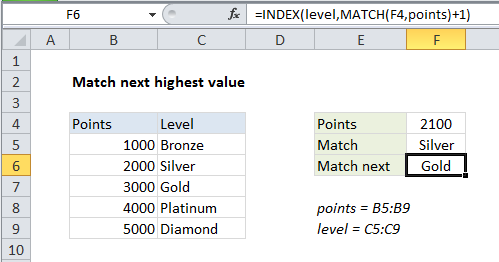## Excel Office

Excel How Tos, Tutorials, Tips & Tricks, Shortcuts

# Match next highest value in Excel

This tutorial shows how to Match next highest value in Excel using the example below;

## Formula

`=INDEX(data,MATCH(lookup,values)+1)`## Explanation

To match the “next highest” value in a lookup table, you can use a formula based on INDEX and MATCH. In the example shown, the formula in F6 is:

`=INDEX(level,MATCH(F4,points)+1)`

where “level” is the named range C5:C9, and “points” is the named range B5:B9.

Worked Example:   Check if range contains a value not in another range in Excel

### How this formula works

This formula is a standard version of INDEX + MATCH with a small twist.

Working from the inside out, MATCH is used find the correct row number for the value in F4, 2100. Without the third argument, match_type, defined, MATCH defaults to approximate match and returns 2.

Worked Example:   Exact match lookup with INDEX and MATCH in Excel

The small twist is that we add 1 to this result to override the matched result and return 3 as the row number for INDEX.

With level (C5:C9) supplied as the array, and 3 as the row number, INDEX returns “Gold”:

`=INDEX(level,3) // returns Gold`

### Another option

The above approach works fine for simple lookups. If you want to use MATCH to find the “next largest” match in more traditional way, you can sort the lookup array in descending order, and use MATCH as described on this page.

Worked Example:   Vlookup Examples in Excel